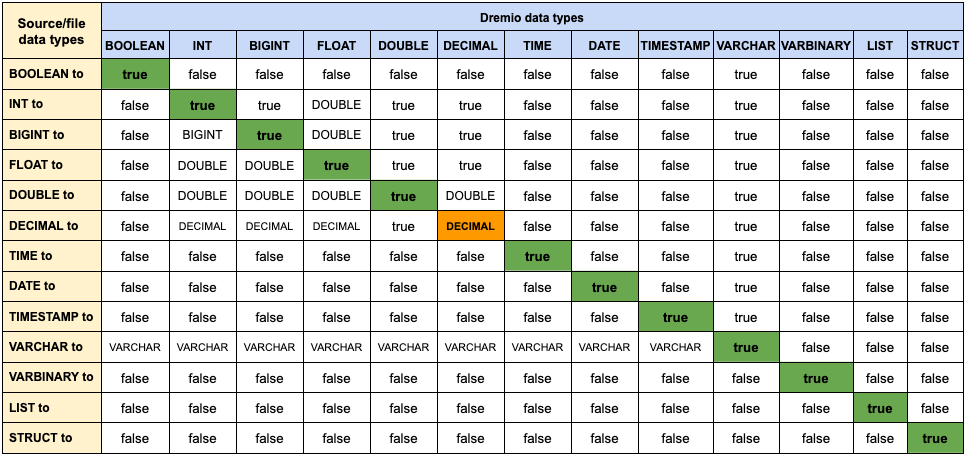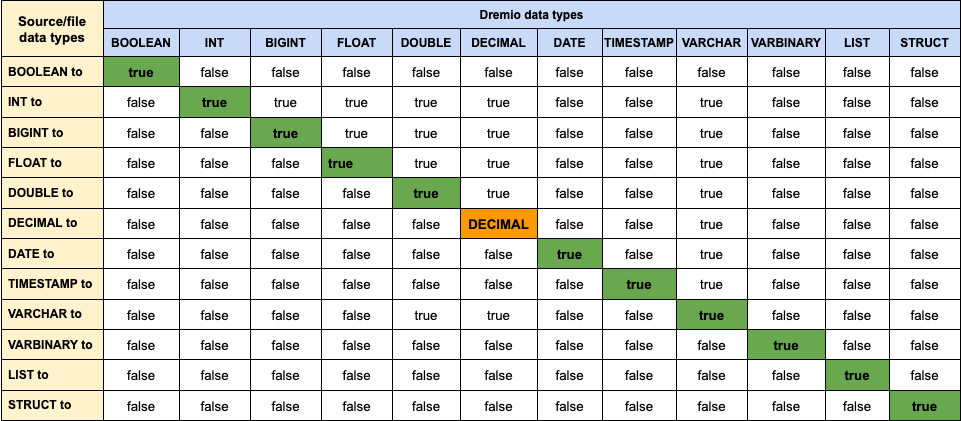Version: current [24.2.x]

# Implicit Coercion of Filesystem Sources

With filesystem sources, you can format a folder so that Dremio treats it as a table. Files within folders store the data of tables. If a data type within a file does not match the data type of the corresponding table, Dremio takes one of the following actions:

1. While reading data files, Dremio coerces the file data type to match the table's schema in Dremio.
2. Dremio performs schema learning, which means that it updates the table's schema to reflect the new data types found in the data files.
3. If the data types cannot be coerced due to belonging to a different data type family, the query fails to run and you receive an error message.

For metastore sources such as AWS Glue, Dremio does not update the data type since the table’s schema is defined by the external metastore. Additionally, for Apache Iceberg and Delta Lake, the table format defines the schema and there is no schema learning.

The following Supported and Unsupported Coercions tables show the data type coercions supported in Dremio and when implicit coercion/conversion can or cannot be performed for file-formatted and AWS Glue sources.

Notes about the Supported and Unsupported Coercions tables:

• The value in a cell identifies the action for the data type:
• True: The data type from the source file is successfully coerced to the Dremio data type.
• Data type indicator: The data type for the column is automatically updated to the stated data type, and the query is rerun. For example, if a column is originally defined as an INT type and Dremio encounters a BIGINT, Dremio will update the data type to BIGINT through schema learning.
• False: No coercion is available and an error is returned.
• VARCHAR: represents STRING

For additional information about the DECIMAL-to-DECIMAL conversion shown in the tables, see DECIMAL-to-DECIMAL Coercions

Table 1: Supported and Unsupported Coercions for File-formatted SourcesTable 2: Supported and Unsupported Coercions for Apache Hive ORC DatasetsFor Apache Hive and AWS Glue sources with Parquet datasets, Dremio supports the following coercions:

• INT to BIGINT
• FLOAT to DOUBLE
• DECIMAL to DECIMAL
• VARCHAR to VARCHAR[N], which truncates VARCHAR to the specified [N] limit

## DECIMAL-to-DECIMAL Coercions​

Since decimals can have different scales and precision, Dremio supports DECIMAL-to-DECIMAL coercions. Decimal coercion support is provided in the following DECIMAL-to-DECIMAL Overflow/Truncation Behavior table.

note

The following symbols are used in the DECIMAL-to-DECIMAL Overflow/Truncation table:

• Precision (p): represents the number of digits in the value/cell.
• Scale (s): represents the number of digits after the decimal point in the value/cell.

Table 3: DECIMAL-to-DECIMAL Overflow/Truncation Behavior

Source/File Data TypeTable SchemaDescriptionOn OverflowOn Truncation
DecimalDecimal[Conversion between any DECIMAL type is supported, including decreasing the `p` or `s`. Checks that are added during runtime help to detect overflow.NullRounds up to the nearest half
note

Implicit conversions/coercions are not currently supported for filesystem and MongoDB sources.

## DECIMAL-to-DECIMAL Schema Learning​

When source files contain decimal values using a different scale or precision from the table’s definition - `Decimal (p, s)` - Dremio will update the table’s decimal definition according to the following logic through schema learning if possible, or otherwise attempt to coerce the source decimal values to the table’s definition.

Logic used for the schema learning option, where `d` = decimal, `p` = precision, and `s` = scale:

• `d1`, `p1`, `s1` - The decimal type in file 1 with a given `p` and `s`
• `d2`, `p2`, `s2` - The decimal type in file 2 with a given `p` and `s`
• Precision - The new precision after schema learning
• Scale - The new scale after schema learning

The following shows the schema-learned decimal type for the column.

Schema learning logic to determine precision
``Precision = max(s1, s2) + max(p1-s1, p2-s2)``
note

This schema provides space for the maximum fractional part (below decimal point) and integral part (above decimal point).

Schema learning logic to determine scale
``Scale = max(s1, s2)``

If the resulting precision is greater than 38, it is reduced to 38, and the corresponding scale is reduced to prevent truncating the integral part:

• Scale is reduced to provide enough space for the integral part. The resulting scale is `MIN(precision, 38) - max(p1 - s1, p2 - s2)`. The resulting precision is 38.
• This result causes truncation on read since the fractional part will not contain enough spaces for the original scale.

## Type Coercion When Copying Data Into Apache Iceberg Tables​

These data-type coercions are supported when the `COPY INTO <table>` SQL command is used to copy data from source files into Iceberg tables:

Source Data TypeTarget Dremio Data Type
StringBIGINT, BOOLEAN, DATE, DECIMAL, DOUBLE, FLOAT, INT, TIME, TIMESTAMP, VARCHAR
IntegerBIGINT, DECIMAL, DOUBLE, FLOAT, INT, VARCHAR
Floating-point numbersDECIMAL, DOUBLE, FLOAT, VARCHAR
BooleanBOOLEAN, VARCHAR
Object (JSON only)STRUCT
Array (JSON only)LIST